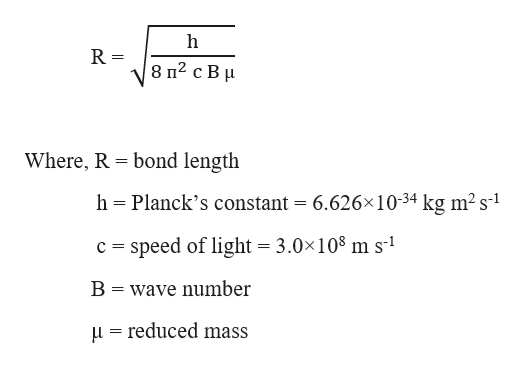# a.) The spacings of the rotational fine structure lines of carbon dioxide 12C16O2 are determined from IR spectroscopy to be 0.7604 cm-1. Calculate the C=O bond length of the molecule (given masses: m(12C) = 12 amu, m(16O) = 15.9949 amu).Ans = ______ Angstromsb.) Suppose that the wavenumber of the J = 1 ← 0 rotational transition of 1H79Br considered as a rigid rotor was measured to be 17.89 cm-1, what is the bond length? Ans = ____  AngstromsWhen the moment of interia is 3.132x10^-47 kg*m^2(Given the isotopic masses:(m(79Br) = 78.9183 amu, m(81Br) = 80.9163 amu)

Question
1 views

a.) The spacings of the rotational fine structure lines of carbon dioxide 12C16O2 are determined from IR spectroscopy to be 0.7604 cm-1. Calculate the C=O bond length of the molecule (given masses: m(12C) = 12 amu, m(16O) = 15.9949 amu).
Ans = ______ Angstroms

b.) Suppose that the wavenumber of the J = 1 ← 0 rotational transition of 1H79Br considered as a rigid rotor was measured to be 17.89 cm-1, what is the bond length? Ans = ____  Angstroms

When the moment of interia is 3.132x10^-47 kg*m^2
(Given the isotopic masses:(m(79Br) = 78.9183 amu, m(81Br) = 80.9163 amu)

check_circle

star
star
star
star
star
1 Rating
Step 1

The bond length of a molecule in IR spectroscopy is calculated using the formula :help_outlineImage Transcriptionclose8 п2 с В и Where, R bond length h Planck's constant = 6.626x10-34 kg m2 s1 c speed of light = 3.0x108 m s1 В wave number ureduced mass fullscreen
Step 2

Given,

Spacing of the rotational fine structure lines, 2B = 0.7604 cm-1

Atomic mass of C, m1 = 12 amu

Atomic mass of O, m2 = 15.9949 amu

The rotational spectra of a diatomic molecule have several peaks spaced by 2B.

Therefore, the value of B can be calculated as:

Step 3

The reduced mass can be...

### Want to see the full answer?

See Solution

#### Want to see this answer and more?

Solutions are written by subject experts who are available 24/7. Questions are typically answered within 1 hour.*

See Solution
*Response times may vary by subject and question.
Tagged in

### Chemistry